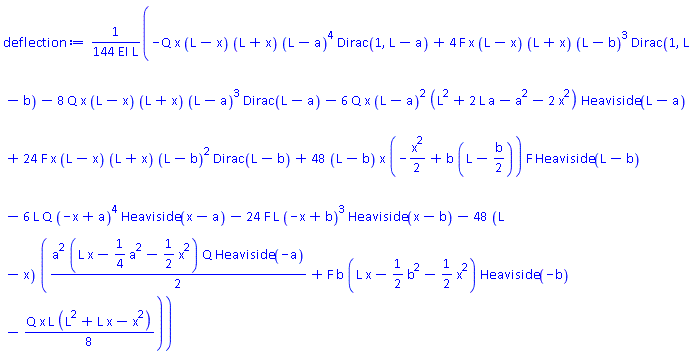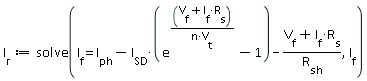Symbolic Math in Maple vs. Mathcad – Maplesoft
Symbolic Math in Mathcad® vs Maple

Both Mathcad and Maple have their own unique design principles. However, Maple’s symbolic math engine is far more powerful, and is allied with an easy-to-use interface.

Here, we describe a few applications of symbolic math in Maple. These are applications for which Mathcad fails to give answers, or simply does not have the functionality to even attempt the analysis.

ODE Solving

Maple offers the world’s most powerful differential equation solvers.

Here, we solve the Euler-Bernoulli equation – a 4th order differential equation - for modeling the bending of a beam.

Euler-Bernoulli equation

$\mathrm{de}≔\mathrm{EI}\cdot \frac{{ⅆ}^{4}}{ⅆ{\mathrm{x}}^{4}}\mathrm{w}\left(\mathrm{x}\right)=\mathrm{q}\left(\mathrm{x}\right):$

Initial and boundary conditions

$\mathrm{ibc}≔\mathrm{w}\left(0\right)=0,\mathrm{w}\left(\mathrm{L}\right)=0,\left(\mathrm{D}@@2\right)\left(\mathrm{w}\right)\left(0\right)=0,\left(\mathrm{D}@@2\right)\left(\mathrm{w}\right)\left(\mathrm{L}\right)=0:$

$\mathrm{q}≔\mathrm{x}\to \mathrm{Q}\cdot \left(1-\mathrm{Heaviside}\left(\mathrm{x}-\mathrm{a}\right)\right)+\mathrm{F}\cdot \mathrm{Dirac}\left(\mathrm{x}-\mathrm{b}\right):$

Solve the differential equation for beam deflection

 $\mathrm{deSol}≔\mathrm{dsolve}\left(\left\{\mathrm{de},\mathrm{ibc}\right\},\mathrm{w}\left(\mathrm{x}\right)\right):\phantom{\rule[-0.0ex]{0.0em}{0.0ex}}\mathrm{deflection}≔\mathrm{simplify}\left(\mathrm{rhs}\left(\mathrm{deSol}\right),\mathrm{symbolic}\right)$Mathcad does not offer any tools for solving ODEs symbolically.

PDE Solving

Independent benchmarks show that Maple is the world’s best tool for solving PDEs.

Here, we solve the heat equation. The answer is in terms of the error function, erf.

${\mathrm{u}}{}\left({\mathrm{x}}{,}{\mathrm{t}}\right){=}\frac{{3}}{{2}}{-}\frac{{\mathrm{erf}}{}\left(\frac{{-}{1}{+}{\mathrm{x}}}{{2}{}\sqrt{{\mathrm{\mu }}}{}\sqrt{{\mathrm{t}}{-}{1}}}\right)}{{2}}{-}{\mathrm{t}}$

Mathcad offers no tools for solving PDEs symbolically.

Solve Equations

Maple has the most powerful symbolic solvers of any math system – here’s why:

Special functions

For example, Maple has a broad database of special functions. These are normally only encountered in advanced mathematical analysis; a few examples are LambertW, Fresnel, Bessel and Appell. Maple lets you use these special functions without any specific training; this helps you rearrange and solve equations that are not possible in Mathcad.

Here, we rearrange the photovoltatic diode equation for the forward current – the result employs the LambertW function.Rearrange complicated trigonometric relationships

Modeling the kinematics of a multibody system often results in complicated trigonometric relationships. Deriving the position constraints means you need to rearrange these equations to give the joint angles. This is a tough problem, especially for more complex systems.

Next, we solve for the position constraints of a 2-DOF robot arm to give the joint angles.

 $\mathrm{posCons}≔\left[\begin{array}{c}\mathrm{cos}\left({\mathrm{\theta }}_{\mathrm{1}}\right)\mathrm{Tx}+\mathrm{sin}\left({\mathrm{\theta }}_{\mathrm{2}}\right)+\mathrm{sin}\left({\mathrm{\theta }}_{\mathrm{1}}\right)\mathrm{Ty}\\ -\mathrm{sin}\left({\mathrm{\theta }}_{\mathrm{1}}\right)\mathrm{Tx}-1-\mathrm{cos}\left({\mathrm{\theta }}_{\mathrm{2}}\right)+\mathrm{cos}\left({\mathrm{\theta }}_{\mathrm{1}}\right)\mathrm{Ty}\end{array}\right]:\phantom{\rule[-0.0ex]{0.0em}{0.0ex}}\mathrm{sol}≔\mathrm{solve}\left(\left\{{\mathrm{posCons}}_{1},{\mathrm{posCons}}_{2}\right\},\left\{{\mathrm{\theta }}_{\mathrm{1}},{\mathrm{\theta }}_{\mathrm{2}}\right\},\mathrm{explicit}\right)\phantom{\rule[-0.0ex]{0.0em}{0.0ex}}\phantom{\rule[-0.0ex]{0.0em}{0.0ex}}$
 ${\mathrm{sol}}{≔}\left\{{{\mathrm{θ}}}_{{\mathrm{1}}}{=}{\mathrm{arctan}}{}\left(\frac{{-}{{\mathrm{Tx}}}^{{2}}{-}{{\mathrm{Ty}}}^{{2}}{+}\frac{\left({{\mathrm{Tx}}}^{{2}}{}{\mathrm{Ty}}{+}{{\mathrm{Ty}}}^{{3}}{+}\sqrt{{-}{{\mathrm{Tx}}}^{{6}}{-}{2}{}{{\mathrm{Tx}}}^{{4}}{}{{\mathrm{Ty}}}^{{2}}{-}{{\mathrm{Tx}}}^{{2}}{}{{\mathrm{Ty}}}^{{4}}{+}{4}{}{{\mathrm{Tx}}}^{{4}}{+}{4}{}{{\mathrm{Tx}}}^{{2}}{}{{\mathrm{Ty}}}^{{2}}}\right){}{\mathrm{Ty}}}{{{\mathrm{Tx}}}^{{2}}{+}{{\mathrm{Ty}}}^{{2}}}}{{\mathrm{Tx}}}{,}\phantom{\rule{0ex}{0ex}}\frac{{{\mathrm{Tx}}}^{{2}}{}{\mathrm{Ty}}{+}{{\mathrm{Ty}}}^{{3}}{+}\sqrt{{-}{{\mathrm{Tx}}}^{{6}}{-}{2}{}{{\mathrm{Tx}}}^{{4}}{}{{\mathrm{Ty}}}^{{2}}{-}{{\mathrm{Tx}}}^{{2}}{}{{\mathrm{Ty}}}^{{4}}{+}{4}{}{{\mathrm{Tx}}}^{{4}}{+}{4}{}{{\mathrm{Tx}}}^{{2}}{}{{\mathrm{Ty}}}^{{2}}}}{{{\mathrm{Tx}}}^{{2}}{+}{{\mathrm{Ty}}}^{{2}}}\right){,}\phantom{\rule{0ex}{0ex}}{{\mathrm{θ}}}_{{\mathrm{2}}}{=}{\mathrm{arctan}}{}\left(\frac{\left({-}{2}{}{{\mathrm{Tx}}}^{{2}}{-}{2}{}{{\mathrm{Ty}}}^{{2}}\right){}\left({{\mathrm{Tx}}}^{{2}}{}{\mathrm{Ty}}{+}{{\mathrm{Ty}}}^{{3}}{+}\sqrt{{-}{{\mathrm{Tx}}}^{{6}}{-}{2}{}{{\mathrm{Tx}}}^{{4}}{}{{\mathrm{Ty}}}^{{2}}{-}{{\mathrm{Tx}}}^{{2}}{}{{\mathrm{Ty}}}^{{4}}{+}{4}{}{{\mathrm{Tx}}}^{{4}}{+}{4}{}{{\mathrm{Tx}}}^{{2}}{}{{\mathrm{Ty}}}^{{2}}}\right)}{{4}{}{\mathrm{Tx}}{}\left({{\mathrm{Tx}}}^{{2}}{+}{{\mathrm{Ty}}}^{{2}}\right)}{+}\frac{{{\mathrm{Tx}}}^{{2}}{}{\mathrm{Ty}}{+}{{\mathrm{Ty}}}^{{3}}}{{2}{}{\mathrm{Tx}}}{,}\frac{{{\mathrm{Tx}}}^{{2}}}{{2}}{+}\frac{{{\mathrm{Ty}}}^{{2}}}{{2}}{-}{1}\right)\right\}{,}\phantom{\rule{0ex}{0ex}}\left\{{{\mathrm{θ}}}_{{\mathrm{1}}}{=}{\mathrm{arctan}}{}\left(\frac{{-}{{\mathrm{Tx}}}^{{2}}{-}{{\mathrm{Ty}}}^{{2}}{-}\frac{\left({-}{{\mathrm{Tx}}}^{{2}}{}{\mathrm{Ty}}{-}{{\mathrm{Ty}}}^{{3}}{+}\sqrt{{-}{{\mathrm{Tx}}}^{{6}}{-}{2}{}{{\mathrm{Tx}}}^{{4}}{}{{\mathrm{Ty}}}^{{2}}{-}{{\mathrm{Tx}}}^{{2}}{}{{\mathrm{Ty}}}^{{4}}{+}{4}{}{{\mathrm{Tx}}}^{{4}}{+}{4}{}{{\mathrm{Tx}}}^{{2}}{}{{\mathrm{Ty}}}^{{2}}}\right){}{\mathrm{Ty}}}{{{\mathrm{Tx}}}^{{2}}{+}{{\mathrm{Ty}}}^{{2}}}}{{2}{}{\mathrm{Tx}}}{,}\phantom{\rule{0ex}{0ex}}{-}\frac{{-}{{\mathrm{Tx}}}^{{2}}{}{\mathrm{Ty}}{-}{{\mathrm{Ty}}}^{{3}}{+}\sqrt{{-}{{\mathrm{Tx}}}^{{6}}{-}{2}{}{{\mathrm{Tx}}}^{{4}}{}{{\mathrm{Ty}}}^{{2}}{-}{{\mathrm{Tx}}}^{{2}}{}{{\mathrm{Ty}}}^{{4}}{+}{4}{}{{\mathrm{Tx}}}^{{4}}{+}{4}{}{{\mathrm{Tx}}}^{{2}}{}{{\mathrm{Ty}}}^{{2}}}}{{2}{}\left({{\mathrm{Tx}}}^{{2}}{+}{{\mathrm{Ty}}}^{{2}}\right)}\right){,}\phantom{\rule{0ex}{0ex}}{=}{\mathrm{arctan}}{}\left({-}\frac{\left({-}{2}{}{{\mathrm{Tx}}}^{{2}}{-}{2}{}{{\mathrm{Ty}}}^{{2}}\right){}\left({-}{{\mathrm{Tx}}}^{{2}}{}{\mathrm{Ty}}{-}{{\mathrm{Ty}}}^{{3}}{+}\sqrt{{-}{{\mathrm{Tx}}}^{{6}}{-}{2}{}{{\mathrm{Tx}}}^{{4}}{}{{\mathrm{Ty}}}^{{2}}{-}{{\mathrm{Tx}}}^{{2}}{}{{\mathrm{Ty}}}^{{4}}{+}{4}{}{{\mathrm{Tx}}}^{{4}}{+}{4}{}{{\mathrm{Tx}}}^{{2}}{}{{\mathrm{Ty}}}^{{2}}}\right)}{{4}{}{\mathrm{Tx}}{}\left({{\mathrm{Tx}}}^{{2}}{+}{{\mathrm{Ty}}}^{{2}}\right)}{+}\frac{{{\mathrm{Tx}}}^{{2}}{}{\mathrm{Ty}}{+}{{\mathrm{Ty}}}^{{3}}}{{2}{}{\mathrm{Tx}}}{,}\frac{{{\mathrm{Tx}}}^{{2}}}{{2}}{+}\frac{{{\mathrm{Ty}}}^{{2}}}{{2}}{-}{1}\right)\right\}$

Mathcad will not rearrange these equations symbolically.

Control Systems Design

Maple offers tools for symbolic control systems modeling. Here, we find the symbolic controllability matrix for a dc motor described by two differential equations.

 $\left[\begin{array}{ccc}\frac{{1}}{{\mathrm{L}}}& {-}\frac{{\mathrm{R}}}{{{\mathrm{L}}}^{{2}}}& \frac{{{\mathrm{R}}}^{{2}}}{{{\mathrm{L}}}^{{3}}}{-}\frac{{{\mathrm{K}}}^{{2}}}{{{\mathrm{L}}}^{{2}}{}{\mathrm{J}}}\\ {0}& {0}& \frac{{\mathrm{K}}}{{\mathrm{J}}{}{\mathrm{L}}}\\ {0}& \frac{{\mathrm{K}}}{{\mathrm{J}}{}{\mathrm{L}}}& {-}\frac{{\mathrm{K}}{}{\mathrm{R}}}{{\mathrm{J}}{}{{\mathrm{L}}}^{{2}}}{-}\frac{{\mathrm{b}}{}{\mathrm{K}}}{{{\mathrm{J}}}^{{2}}{}{\mathrm{L}}}\end{array}\right]$

Mathcad offers no tools for symbolic control systems design.

Code Generation

Maple can generate C code from symbolic math results. Maple factors out common subexpressions; this makes the code far more numerically efficient than hand-written code.

 t1 = Tx * Tx; t2 = Ty * Ty; t5 = t1 * t1; t9 = t2 * t2; t15 = sqrt(0.4e1 * t2 * t1 - t5 * t1 - t9 * t1 - 0.2e1 * t2 * t5 + 0.4e1 * t5); t16 = Ty * t1 + t2 * Ty + t15; t19 = 0.1e1 / (t1 + t2); t25 = atan2(0.1e1 / Tx * (t19 * Ty * t16 - t1 - t2), t19 * t16);

Mathcad has no code translation tools.

Prêt(e) pour l'étape suivante ?

*Ce programme d'évaluation n'est proposé actuellement ni aux étudiants ni aux particuliers.

Achetez: